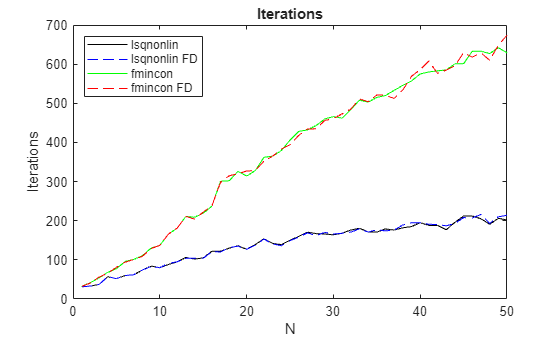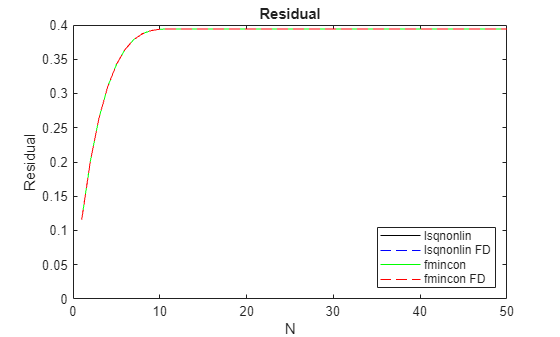# Compare `lsqnonlin` and `fmincon` for Constrained Nonlinear Least Squares

This example shows that `lsqnonlin` generally takes fewer function evaluations than `fmincon` when solving constrained least-squares problems. Both solvers use the `fmincon` `'interior-point'` algorithm for solving the problem. Yet `lsqnonlin` typically solves problems in fewer function evaluations. The reason is that `lsqnonlin` has more information to work with. `fmincon` minimizes the sum of squares given as $\sum _{i}{F}_{i}^{2}$, where $F$ is a vector function. In contrast, `lsqnonlin` works with the entire vector $F$, meaning it has access to all the components of the sum. In other words, `fmincon` can access only the value of the sum, but `lsqnonlin` can access each component separately.

The `runlsqfmincon` helper function listed at the end of this example creates a series of scaled Rosenbrock-type problems with nonlinear constraints for $N$ ranging from 1 to 50, where the number of problem variables is $2N$. For a description of the Rosenbrock function, see Solve a Constrained Nonlinear Problem, Problem-Based. The function also plots the results, showing:

• Number of iterations

• Number of function counts

• Resulting residuals

The plots show the differences between finite-difference derivative estimates (labeled FD) and derivatives calculated using automatic differentiation. For a description of automatic differentiation, see Automatic Differentiation Background.

`runlsqfmincon;`The plots show the following results, which are typical.

• For each $N$, the number of iterations for `fmincon` is more than double that of `lsqnonlin` and increases approximately linearly with $N$.

• The number of iterations does not depend on the derivative estimation scheme.

• The function count for finite difference (FD) estimation is much higher than for automatic differentiation.

• The function count for `lsqnonlin` is lower than that for `fmincon` for the same derivative estimation scheme.

• The residuals match for all solution methods, meaning the results are independent of the solver and derivative estimation scheme.

The results indicate that `lsqnonlin` is more efficient than `fmincon` in terms of both iterations and function counts. However, different problems can have different results, and for some problems `fmincon` is more efficient than `lsqnonlin`.

### Helper Function

This code creates the `runlsqfmincon` helper function.

```function [lsq,lsqfd,fmin,fminfd] = runlsqfmincon() optslsq = optimoptions("lsqnonlin",Display="none",... MaxFunctionEvaluations=1e5,MaxIterations=1e4); % Allow for many iterations and Fevals optsfmincon = optimoptions("fmincon",Display="none",... MaxFunctionEvaluations=1e5,MaxIterations=1e4); % Create structures to hold results z = zeros(1,50); lsq = struct('Iterations',z,'Fcount',z,'Residual',z); lsqfd = lsq; fmin = lsq; fminfd = lsq; rng(1) % Reproducible initial points x00 = -1/2 + randn(50,1); y00 = 1/2 + randn(50,1); for N = 1:50 x = optimvar("x",N,LowerBound=-3,UpperBound=3); y = optimvar("y",N,LowerBound=0,UpperBound=9); prob = optimproblem("Objective",sum((10*(y - x.^2)).^2 + (1 - x).^2)); x0.x = x00(1:N); x0.y = y00(1:N); % Include a set of nonlinear inequality constraints cons = optimconstr(N); for i = 1:N cons(i) = x(i)^2 + y(i)^2 <= 1/2 + 1/8*i; end prob.Constraints = cons; [sol,fval,exitflag,output] = solve(prob,x0,Options=optslsq); lsq.Iterations(N) = output.iterations; lsq.Fcount(N) = output.funcCount; lsq.Residual(N) = fval; [sol,fval,exitflag,output] = solve(prob,x0,Options=optslsq,... ObjectiveDerivative='finite-differences',ConstraintDerivative='finite-differences'); lsqfd.Iterations(N) = output.iterations; lsqfd.Fcount(N) = output.funcCount; lsqfd.Residual(N) = fval; [sol,fval,exitflag,output] = solve(prob,x0,Options=optsfmincon,Solver="fmincon"); fmin.Iterations(N) = output.iterations; fmin.Fcount(N) = output.funcCount; fmin.Residual(N) = fval; [sol,fval,exitflag,output] = solve(prob,x0,Options=optsfmincon,Solver="fmincon",... ObjectiveDerivative='finite-differences',ConstraintDerivative='finite-differences'); fminfd.Iterations(N) = output.iterations; fminfd.Fcount(N) = output.funcCount; fminfd.Residual(N) = fval; end N = 1:50; plot(N,lsq.Iterations,'k',N,lsqfd.Iterations,'b--',N,fmin.Iterations,'g',N,fminfd.Iterations,'r--') legend('lsqnonlin','lsqnonlin FD','fmincon','fmincon FD','Location','northwest') xlabel('N') ylabel('Iterations') title('Iterations') figure semilogy(N,lsq.Fcount,'k',N,lsqfd.Fcount,'b--',N,fmin.Fcount,'g',N,fminfd.Fcount,'r--') legend('lsqnonlin','lsqnonlin FD','fmincon','fmincon FD','Location','northwest') xlabel('N') ylabel('log(Function count)') title('Function count, log-scaled') figure plot(N,lsq.Residual,'k',N,lsqfd.Residual,'b--',N,fmin.Residual,'g',N,fminfd.Residual,'r--') legend('lsqnonlin','lsqnonlin FD','fmincon','fmincon FD','Location','southeast') xlabel('N') ylabel('Residual') ylim([0,0.4]) title('Residual') end```